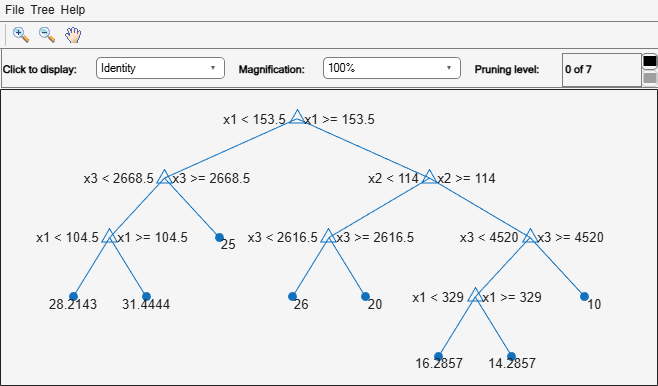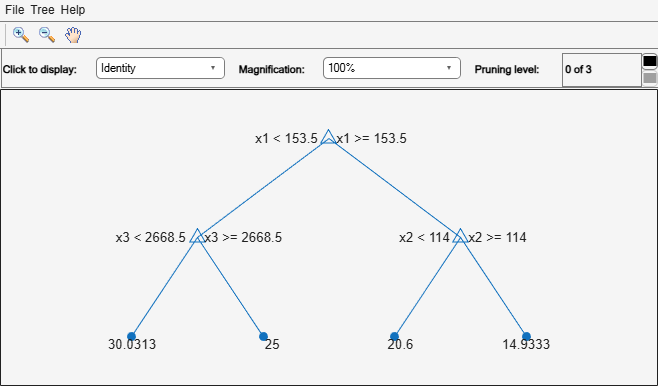# resubLoss

Class: RegressionTree

Regression error by resubstitution

## Syntax

```L = resubLoss(tree) L = resubLoss(tree,Name,Value) L = resubLoss(tree,'Subtrees',subtreevector) [L,se] = resubLoss(tree,'Subtrees',subtreevector) [L,se,NLeaf] = resubLoss(tree,'Subtrees',subtreevector) [L,se,NLeaf,bestlevel] = resubLoss(tree,'Subtrees',subtreevector) [L,...] = resubLoss(tree,'Subtrees',subtreevector,Name,Value) ```

## Description

`L = resubLoss(tree)` returns the resubstitution loss, meaning the loss computed for the data that `fitrtree` used to create `tree`.

`L = resubLoss(tree,Name,Value)` returns the loss with additional options specified by one or more `Name,Value` pair arguments. You can specify several name-value pair arguments in any order as `Name1,Value1,…,NameN,ValueN`.

`L = resubLoss(tree,'Subtrees',subtreevector)` returns a vector of mean squared errors for the trees in the pruning sequence `subtreevector`.

```[L,se] = resubLoss(tree,'Subtrees',subtreevector)``` returns the vector of standard errors of the classification errors.

```[L,se,NLeaf] = resubLoss(tree,'Subtrees',subtreevector)``` returns the vector of numbers of leaf nodes in the trees of the pruning sequence.

```[L,se,NLeaf,bestlevel] = resubLoss(tree,'Subtrees',subtreevector)``` returns the best pruning level as defined in the `TreeSize` name-value pair. By default, `bestlevel` is the pruning level that gives loss within one standard deviation of minimal loss.

`[L,...] = resubLoss(tree,'Subtrees',subtreevector,Name,Value)` returns loss statistics with additional options specified by one or more `Name,Value` pair arguments. You can specify several name-value pair arguments in any order as `Name1,Value1,…,NameN,ValueN`.

## Input Arguments

expand all

A regression tree (`RegressionTree` model object) constructed using `fitrtree`.

### Name-Value Pair Arguments

Specify optional comma-separated pairs of `Name,Value` arguments. `Name` is the argument name and `Value` is the corresponding value. `Name` must appear inside quotes. You can specify several name and value pair arguments in any order as `Name1,Value1,...,NameN,ValueN`.

Loss function, specified as a function handle or `'mse'` meaning mean squared error.

You can write your own loss function in the syntax described in Loss Functions.

Data Types: `char` | `string` | `function_handle`

`Name,Value` arguments associated with pruning subtrees:

Pruning level, specified as the comma-separated pair consisting of `'Subtrees'` and a vector of nonnegative integers in ascending order or `'all'`.

If you specify a vector, then all elements must be at least `0` and at most `max(tree.PruneList)`. `0` indicates the full, unpruned tree and `max(tree.PruneList)` indicates the completely pruned tree (i.e., just the root node).

If you specify `'all'`, then `resubLoss` operates on all subtrees (i.e., the entire pruning sequence). This specification is equivalent to using `0:max(tree.PruneList)`.

`resubLoss` prunes `tree` to each level indicated in `Subtrees`, and then estimates the corresponding output arguments. The size of `Subtrees` determines the size of some output arguments.

To invoke `Subtrees`, the properties `PruneList` and `PruneAlpha` of `tree` must be nonempty. In other words, grow `tree` by setting `'Prune','on'`, or by pruning `tree` using `prune`.

Example: `'Subtrees','all'`

Data Types: `single` | `double` | `char` | `string`

Tree size, specified as one of the following:

• `'se'``loss` returns the highest pruning level with loss within one standard deviation of the minimum (`L` + `se`, where `L` and `se` relate to the smallest value in `Subtrees`).

• `'min'``loss` returns the element of `Subtrees` with smallest loss, usually the smallest element of `Subtrees`.

## Output Arguments

expand all

Regression loss (mean squared error), a vector the length of `Subtrees`. The meaning of the error depends on the values in `Weights` and `LossFun`.

Standard error of loss, a vector the length of `Subtrees`.

Number of leaves (terminal nodes) in the pruned subtrees, a vector the length of `Subtrees`.

A scalar whose value depends on `TreeSize`:

• `TreeSize` = `'se'``loss` returns the highest pruning level with loss within one standard deviation of the minimum (`L` + `se`, where `L` and `se` relate to the smallest value in `Subtrees`).

• `TreeSize` = `'min'``loss` returns the element of `Subtrees` with smallest loss, usually the smallest element of `Subtrees`.

## Examples

expand all

Load the `carsmall` data set. Consider `Displacement`, `Horsepower`, and `Weight` as predictors of the response `MPG`.

```load carsmall X = [Displacement Horsepower Weight];```

Grow a regression tree using all observations.

`Mdl = fitrtree(X,MPG);`

Compute the resubstitution MSE.

`resubLoss(Mdl)`
```ans = 4.8952 ```

Unpruned decision trees tend to overfit. One way to balance model complexity and out-of-sample performance is to prune a tree (or restrict its growth) so that in-sample and out-of-sample performance are satisfactory.

Load the `carsmall` data set. Consider `Displacement`, `Horsepower`, and `Weight` as predictors of the response `MPG`.

```load carsmall X = [Displacement Horsepower Weight]; Y = MPG;```

Partition the data into training (50%) and validation (50%) sets.

```n = size(X,1); rng(1) % For reproducibility idxTrn = false(n,1); idxTrn(randsample(n,round(0.5*n))) = true; % Training set logical indices idxVal = idxTrn == false; % Validation set logical indices```

Grow a regression tree using the training set.

`Mdl = fitrtree(X(idxTrn,:),Y(idxTrn));`

View the regression tree.

`view(Mdl,'Mode','graph');`The regression tree has seven pruning levels. Level 0 is the full, unpruned tree (as displayed). Level 7 is just the root node (i.e., no splits).

Examine the training sample MSE for each subtree (or pruning level) excluding the highest level.

```m = max(Mdl.PruneList) - 1; trnLoss = resubLoss(Mdl,'SubTrees',0:m)```
```trnLoss = 7×1 5.9789 6.2768 6.8316 7.5209 8.3951 10.7452 14.8445 ```
• The MSE for the full, unpruned tree is about 6 units.

• The MSE for the tree pruned to level 1 is about 6.3 units.

• The MSE for the tree pruned to level 6 (i.e., a stump) is about 14.8 units.

Examine the validation sample MSE at each level excluding the highest level.

`valLoss = loss(Mdl,X(idxVal,:),Y(idxVal),'SubTrees',0:m)`
```valLoss = 7×1 32.1205 31.5035 32.0541 30.8183 26.3535 30.0137 38.4695 ```
• The MSE for the full, unpruned tree (level 0) is about 32.1 units.

• The MSE for the tree pruned to level 4 is about 26.4 units.

• The MSE for the tree pruned to level 5 is about 30.0 units.

• The MSE for the tree pruned to level 6 (i.e., a stump) is about 38.5 units.

To balance model complexity and out-of-sample performance, consider pruning `Mdl` to level 4.

```pruneMdl = prune(Mdl,'Level',4); view(pruneMdl,'Mode','graph')```